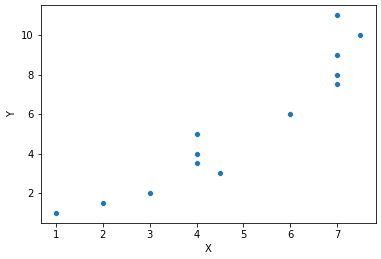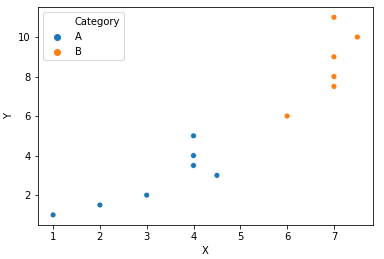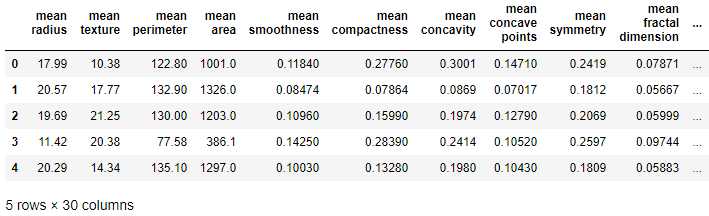# Support Vector Machines (SVM) – Step-by-Step guide

In this post, we will briefly take a look at the Support Vector Machines Machine learning algorithm.

## Overview

Support Vector Machines shortly referred to as SVM is a supervised machine learning algorithm.

It is mostly used for classification problems.

## Support Vector Machine Algorithm

### Plotting the points in n-dimensional space

This algorithm works by plotting each point on an n-dimensional space.

It is difficult to imagine the hyperplanes in more than 2-dimensional space. Hence, we will understand the algorithm by just plotting the points in a 2-dimensional space itself.

First of all, the algorithm will plot the points.### Point segregation

After plotting the points, the algorithm will segregate the points based on the co-ordinates.### Hyperplane possibilities

Based on the position of the points in the n-dimensional space, a hyperplane will segregate the plotted points into groups.But, there are a lot many hyperplanes that can separate the group of points in the n-dimensional space.### Hyperplane with maximum margin

But, the SVM algorithm will ensure that the hyperplane which separates the points is with the maximum margin.

That is, the distance between the two groups of points, which is referred to as margin is the maximum.Just take a look at the above picture.

The Y plane maintains the maximum distance between both the group of points. Hence, plane Y is rightly selected to be the segregating plane between the group of points.

### Multi-dimensional spaces in SVM

What we saw above is only in a 2-dimensional space. The reason is we had only two attributes to the data X and Y.

But, the reality is, when we have several attributes(columns) in the data, that many-dimensional space is constructed which is hard to imagine.

### Prediction

To predict a new value.

The algorithm will rightly plot the new value into the n-dimensional system. And finds out to which category the new point belongs to. That’s all.

## Support Vector Machines on Cancer dataset

### Import necessary modules

```import pandas as pd
import numpy as np

import matplotlib.pyplot as plt
import seaborn as sns
%matplotlib inline
```

### Import dataset

```from sklearn.datasets import load_breast_cancer

```

Now, the dataset is loaded into the variable “cancer”.

### Keys of the dataset

Let us explore the keys of the cancer dataset.

```cancer.keys()
```
Out:
```dict_keys(['data', 'target', 'target_names',
'DESCR', 'feature_names'])```

### Dataframe construction

```df_feat = pd.DataFrame(cancer['data'],
columns = cancer['feature_names'])
```

We will convert the data into a pandas Dataframe with the name “df_feat”.

```df_feat.head()
```The dataframe has 30 columns in total.

### Train Test split

Now, we will split the data into training and testing datasets. First of all, we will import train_test_split from Scikit-learn(sklearn).

```from sklearn.cross_validation import train_test_split
```

Now, we will assign the X and y values. X values are the 30 columns shown above. y value corresponds to the target.

```X = df_feat
y = cancer['target']
```

We will split the data into the training set and testing set.

```X_train, X_test, y_train, y_test = train_test_split(X,
y, test_size=0.33)
```

### Support Vector Machine model import

```from sklearn.svm import SVC
model = SVC()
```

### Fitting the model with the training set

We have our training sets X_train and y_train. We will fit these training datasets into our model.

```model.fit(X_train, y_train)
```
```SVC(C=1.0, cache_size=200, class_weight=None, coef0=0.0,
decision_function_shape='ovr', degree=3, gamma='auto', kernel='rbf',
max_iter=-1, probability=False, random_state=None, shrinking=True,
tol=0.001, verbose=False)```

### Predicting the target for the testing set

Now, we need to evaluate whether our data is performing perfectly by using our testing dataset.

```predictions = model.predict(X_test)
```

### Confusion Matrix and Classification Report

We will take a look at the performance parameters of our SVM model.

```print(confusion_matrix(y_test, predictions))
print('\n')
print(classification_report(y_test, predictions))
```
```[[  0  66]
[  0 122]]

precision    recall  f1-score   support

0       0.00      0.00      0.00        66
1       0.65      1.00      0.79       122

avg / total       0.42      0.65      0.51       188```

Ooops… unfortunately our model is performing poorly. The precision is just 42% which is very bad.

Just take a look at the code output in the section “Fitting the model with the training set“. In the output, the model parameters such as C and gamma are given default values(1 and ‘auto’ respectively). If we can vary the C and gamma values we can easily increase the performance parameters of our model.

But, how do we know which value of C and gamma will provide the maximum precision and accuracy?

## Grid Search in Support Vector Machine

Grid search will try all possible values for the model attributes. We can easily figure out the optimum value of model attributes such as C and gamma that provide the highest model performance.

Let’s look at how to perform a grid search.

### Importing GridSearch

We will import the inbuilt grid search functionality from Scikit-learn.

```from sklearn.grid_search import GridSearchCV
```

### Parameter grid construction

Our grid search functionality requires parameters to try it on the model and evaluate its performance. Hence, it is important for us to construct a parameter grid.

The parameter grid we are about to create a Python dictionary.

```param_grid = {'C':[0.1, 1, 10, 100, 1000],
'gamma':[1, 0.1, 0.01, 0.001, 0.0001]}
```

### Grid definition

We will define the grid with our SVC model.

```grid = GridSearchCV(SVC(), param_grid, verbose = 3)
```

### Fitting the grid with the training set

Let’s fit our grid with the training dataset.

```grid.fit(X_train, y_train)
```

And you will have a lengthy output with all possible parameter combinations.

```Fitting 3 folds for each of 25 candidates, totalling 75 fits
[CV] C=0.1, gamma=1 ..................................................
[CV] ......................... C=0.1, gamma=1, score=0.637795 -   0.0s
[CV] C=0.1, gamma=1 ..................................................
[CV] ......................... C=0.1, gamma=1, score=0.637795 -   0.0s
[CV] C=0.1, gamma=1 ..................................................
[CV] ......................... C=0.1, gamma=1, score=0.637795 -   0.0s
[CV] C=0.1, gamma=0.1 ................................................
[CV] ....................... C=0.1, gamma=0.1, score=0.637795 -   0.0s
[CV] C=0.1, gamma=0.1 ................................................
[CV] ....................... C=0.1, gamma=0.1, score=0.637795 -   0.0s
[CV] C=0.1, gamma=0.1 ................................................
[CV] ....................... C=0.1, gamma=0.1, score=0.637795 -   0.0s
[CV] C=0.1, gamma=0.01 ...............................................
[CV] ...................... C=0.1, gamma=0.01, score=0.637795 -   0.0s
[CV] C=0.1, gamma=0.01 ...............................................
[CV] ...................... C=0.1, gamma=0.01, score=0.637795 -   0.0s
[CV] C=0.1, gamma=0.01 ...............................................
[CV] ...................... C=0.1, gamma=0.01, score=0.637795 -   0.0s
[CV] C=0.1, gamma=0.001 ..............................................
[CV] ..................... C=0.1, gamma=0.001, score=0.637795 -   0.0s
[CV] C=0.1, gamma=0.001 ..............................................
[CV] ..................... C=0.1, gamma=0.001, score=0.637795 -   0.0s
[CV] C=0.1, gamma=0.001 ..............................................
```
```[Parallel(n_jobs=1)]: Done   1 out of   1 | elapsed:    0.0s remaining:    0.0s
[Parallel(n_jobs=1)]: Done   2 out of   2 | elapsed:    0.0s remaining:    0.0s
```
```[CV] ..................... C=0.1, gamma=0.001, score=0.637795 -   0.0s
[CV] C=0.1, gamma=0.0001 .............................................
[CV] .................... C=0.1, gamma=0.0001, score=0.921260 -   0.0s
[CV] C=0.1, gamma=0.0001 .............................................
[CV] .................... C=0.1, gamma=0.0001, score=0.874016 -   0.0s
[CV] C=0.1, gamma=0.0001 .............................................
[CV] .................... C=0.1, gamma=0.0001, score=0.929134 -   0.0s
[CV] C=1, gamma=1 ....................................................
[CV] ........................... C=1, gamma=1, score=0.637795 -   0.0s
[CV] C=1, gamma=1 ....................................................
[CV] ........................... C=1, gamma=1, score=0.637795 -   0.0s
[CV] C=1, gamma=1 ....................................................
[CV] ........................... C=1, gamma=1, score=0.637795 -   0.0s
[CV] C=1, gamma=0.1 ..................................................
[CV] ......................... C=1, gamma=0.1, score=0.637795 -   0.0s
[CV] C=1, gamma=0.1 ..................................................
[CV] ......................... C=1, gamma=0.1, score=0.637795 -   0.0s
[CV] C=1, gamma=0.1 ..................................................
[CV] ......................... C=1, gamma=0.1, score=0.637795 -   0.0s
[CV] C=1, gamma=0.01 .................................................
[CV] ........................ C=1, gamma=0.01, score=0.637795 -   0.0s
[CV] C=1, gamma=0.01 .................................................
[CV] ........................ C=1, gamma=0.01, score=0.637795 -   0.0s
[CV] C=1, gamma=0.01 .................................................
[CV] ........................ C=1, gamma=0.01, score=0.637795 -   0.0s
[CV] C=1, gamma=0.001 ................................................
[CV] ....................... C=1, gamma=0.001, score=0.921260 -   0.0s
[CV] C=1, gamma=0.001 ................................................
[CV] ....................... C=1, gamma=0.001, score=0.874016 -   0.0s
[CV] C=1, gamma=0.001 ................................................
[CV] ....................... C=1, gamma=0.001, score=0.913386 -   0.0s
[CV] C=1, gamma=0.0001 ...............................................
[CV] ...................... C=1, gamma=0.0001, score=0.937008 -   0.0s
[CV] C=1, gamma=0.0001 ...............................................
[CV] ...................... C=1, gamma=0.0001, score=0.905512 -   0.0s
[CV] C=1, gamma=0.0001 ...............................................
[CV] ...................... C=1, gamma=0.0001, score=0.921260 -   0.0s
[CV] C=10, gamma=1 ...................................................
[CV] .......................... C=10, gamma=1, score=0.637795 -   0.0s
[CV] C=10, gamma=1 ...................................................
[CV] .......................... C=10, gamma=1, score=0.637795 -   0.0s
[CV] C=10, gamma=1 ...................................................
[CV] .......................... C=10, gamma=1, score=0.637795 -   0.0s
[CV] C=10, gamma=0.1 .................................................
[CV] ........................ C=10, gamma=0.1, score=0.637795 -   0.0s
[CV] C=10, gamma=0.1 .................................................
[CV] ........................ C=10, gamma=0.1, score=0.637795 -   0.0s
[CV] C=10, gamma=0.1 .................................................
[CV] ........................ C=10, gamma=0.1, score=0.637795 -   0.0s
[CV] C=10, gamma=0.01 ................................................
[CV] ....................... C=10, gamma=0.01, score=0.637795 -   0.0s
[CV] C=10, gamma=0.01 ................................................
[CV] ....................... C=10, gamma=0.01, score=0.645669 -   0.0s
[CV] C=10, gamma=0.01 ................................................
[CV] ....................... C=10, gamma=0.01, score=0.637795 -   0.0s
[CV] C=10, gamma=0.001 ...............................................
[CV] ...................... C=10, gamma=0.001, score=0.905512 -   0.0s
[CV] C=10, gamma=0.001 ...............................................
[CV] ...................... C=10, gamma=0.001, score=0.889764 -   0.0s
[CV] C=10, gamma=0.001 ...............................................
[CV] ...................... C=10, gamma=0.001, score=0.905512 -   0.0s
[CV] C=10, gamma=0.0001 ..............................................
[CV] ..................... C=10, gamma=0.0001, score=0.960630 -   0.0s
[CV] C=10, gamma=0.0001 ..............................................
[CV] ..................... C=10, gamma=0.0001, score=0.913386 -   0.0s
[CV] C=10, gamma=0.0001 ..............................................
[CV] ..................... C=10, gamma=0.0001, score=0.921260 -   0.0s
[CV] C=100, gamma=1 ..................................................
[CV] ......................... C=100, gamma=1, score=0.637795 -   0.0s
[CV] C=100, gamma=1 ..................................................
[CV] ......................... C=100, gamma=1, score=0.637795 -   0.0s
[CV] C=100, gamma=1 ..................................................
[CV] ......................... C=100, gamma=1, score=0.637795 -   0.0s
[CV] C=100, gamma=0.1 ................................................
[CV] ....................... C=100, gamma=0.1, score=0.637795 -   0.0s
[CV] C=100, gamma=0.1 ................................................
[CV] ....................... C=100, gamma=0.1, score=0.637795 -   0.0s
[CV] C=100, gamma=0.1 ................................................
[CV] ....................... C=100, gamma=0.1, score=0.637795 -   0.0s
[CV] C=100, gamma=0.01 ...............................................
[CV] ...................... C=100, gamma=0.01, score=0.637795 -   0.0s
[CV] C=100, gamma=0.01 ...............................................
[CV] ...................... C=100, gamma=0.01, score=0.645669 -   0.0s
[CV] C=100, gamma=0.01 ...............................................
[CV] ...................... C=100, gamma=0.01, score=0.637795 -   0.0s
[CV] C=100, gamma=0.001 ..............................................
[CV] ..................... C=100, gamma=0.001, score=0.905512 -   0.0s
[CV] C=100, gamma=0.001 ..............................................
[CV] ..................... C=100, gamma=0.001, score=0.889764 -   0.0s
[CV] C=100, gamma=0.001 ..............................................
[CV] ..................... C=100, gamma=0.001, score=0.905512 -   0.0s
[CV] C=100, gamma=0.0001 .............................................
[CV] .................... C=100, gamma=0.0001, score=0.929134 -   0.0s
[CV] C=100, gamma=0.0001 .............................................
[CV] .................... C=100, gamma=0.0001, score=0.921260 -   0.0s
[CV] C=100, gamma=0.0001 .............................................
[CV] .................... C=100, gamma=0.0001, score=0.889764 -   0.0s
[CV] C=1000, gamma=1 .................................................
[CV] ........................ C=1000, gamma=1, score=0.637795 -   0.0s
[CV] C=1000, gamma=1 .................................................
[CV] ........................ C=1000, gamma=1, score=0.637795 -   0.0s
[CV] C=1000, gamma=1 .................................................
[CV] ........................ C=1000, gamma=1, score=0.637795 -   0.0s
[CV] C=1000, gamma=0.1 ...............................................
[CV] ...................... C=1000, gamma=0.1, score=0.637795 -   0.0s
[CV] C=1000, gamma=0.1 ...............................................
[CV] ...................... C=1000, gamma=0.1, score=0.637795 -   0.0s
[CV] C=1000, gamma=0.1 ...............................................
[CV] ...................... C=1000, gamma=0.1, score=0.637795 -   0.0s
[CV] C=1000, gamma=0.01 ..............................................
[CV] ..................... C=1000, gamma=0.01, score=0.637795 -   0.0s
[CV] C=1000, gamma=0.01 ..............................................
[CV] ..................... C=1000, gamma=0.01, score=0.645669 -   0.0s
[CV] C=1000, gamma=0.01 ..............................................
[CV] ..................... C=1000, gamma=0.01, score=0.637795 -   0.0s
[CV] C=1000, gamma=0.001 .............................................
[CV] .................... C=1000, gamma=0.001, score=0.905512 -   0.0s
[CV] C=1000, gamma=0.001 .............................................
[CV] .................... C=1000, gamma=0.001, score=0.889764 -   0.0s
[CV] C=1000, gamma=0.001 .............................................
[CV] .................... C=1000, gamma=0.001, score=0.905512 -   0.0s
[CV] C=1000, gamma=0.0001 ............................................
```
```[CV] ................... C=1000, gamma=0.0001, score=0.937008 -   0.0s
[CV] C=1000, gamma=0.0001 ............................................
[CV] ................... C=1000, gamma=0.0001, score=0.905512 -   0.0s
[CV] C=1000, gamma=0.0001 ............................................
[CV] ................... C=1000, gamma=0.0001, score=0.889764 -   0.0s
```
```[Parallel(n_jobs=1)]: Done  75 out of  75 | elapsed:    1.2s finished
```
Out:
```GridSearchCV(cv=None, error_score='raise',
estimator=SVC(C=1.0, cache_size=200, class_weight=None, coef0=0.0,
decision_function_shape='ovr', degree=3, gamma='auto', kernel='rbf',
max_iter=-1, probability=False, random_state=None, shrinking=True,
tol=0.001, verbose=False),
fit_params={}, iid=True, n_jobs=1,
param_grid={'C': [0.1, 1, 10, 100, 1000], 'gamma': [1, 0.1, 0.01, 0.001, 0.0001]},
pre_dispatch='2*n_jobs', refit=True, scoring=None, verbose=3)

```

### Finding the best parameter combination

It is tough to navigate through the above output to find out which C-gamma parameter combination had the maximum score.

```grid.best_params_
```
`{'C': 10, 'gamma': 0.0001}`

Also, we can find out the best combination of all the parameters.

```grid.best_estimator_
```
```SVC(C=10, cache_size=200, class_weight=None, coef0=0.0,
decision_function_shape='ovr', degree=3, gamma=0.0001, kernel='rbf',
max_iter=-1, probability=False, random_state=None, shrinking=True,
tol=0.001, verbose=False)```

### Prediction

```grid_predictions = grid.predict(X_test)
```

### Performance Evaluation

Let’s take a look at the confusion matrix and the classification report.

```print(confusion_matrix(y_test, grid_predictions))
print('\n')
print(classification_report(y_test, grid_predictions))
```
```[[ 64  10]
[  4 110]]

precision    recall  f1-score   support

0       0.94      0.86      0.90        74
1       0.92      0.96      0.94       114

avg / total       0.93      0.93      0.92       188```

There is a drastic improvement in precision and other parameters. All because of using the grid search and finding the best parameter combinations.

Now, our Support Vector Machines model is decent enough to predict whether a case is cancerous or not. 🙂